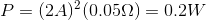# AP Physics C: Mechanics : Circuit Relationships

## Example Questions

### Example Question #15 : Electric Circuits

Three resistors R1, R2, and R3 and the capacitor, C, are connected to an ideal battery V to complete the circuit as shown.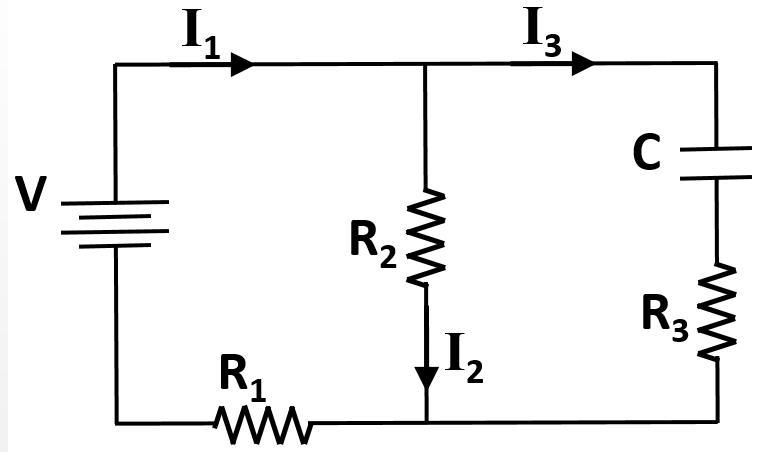After the circuit has been connected for a very long time, the currents in each branch of the circuit are measured to be some values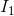,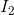, and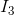, and the charge on the capacitor some value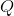.

Which of the following equations is a correct expression regarding the voltage of the circuit after a long time?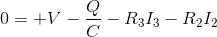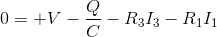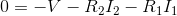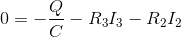Explanation:

Identifying the sum of the voltage drops and rises (Kirchoff's Loop Law) around the three possible loops of this circuit is the key to answering this question correctly. The following signs can be assigned to each of the circuit elements based on the direction of the currents given.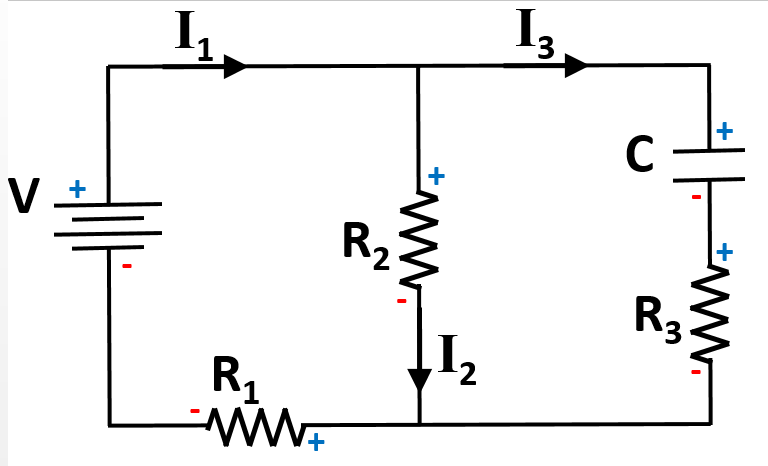Use Ohm's law and the equation for capacitance to derive terms for the voltage across each element of the circuit.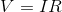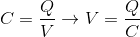There are three possible paths through the circuit, resulting in three correct equations that could be derived: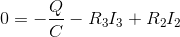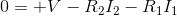Only one of the given answer options matches up correctly to these.

### Example Question #11 : Electric Circuits

A simple circuit consists of a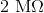resistor that's connected to a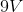battery. How much power is dissipated by the resistor?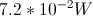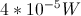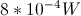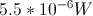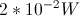Explanation:

One of the formulas for power is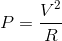. We are given the values of the voltage and resistance.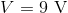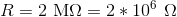Using these values, we can solve for power.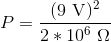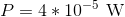### Example Question #1 : Circuit Relationships

A cicuit with a voltage source of 40V has a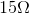resistor and a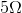resistor in series, followed by tworesistors in parallel. After the parallel branches rejoin, there is a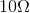resistor before the circuit is closed at the voltage source.

What is the current supplied by the source?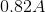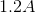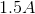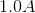Explanation:

The current supplied by the source can be calculated using a derivation of Ohm's law: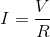Start by finding the equivalent resistance of the circuit.

Sum the first two resistors in series: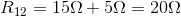Calculate the equivalent resistance of the two resistors in parallel: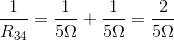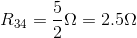Now, all of the reistances can be viewed as being in series.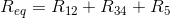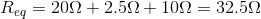Returning to our current calulation, we can find our final answer: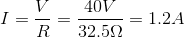### Example Question #18 : Electric Circuits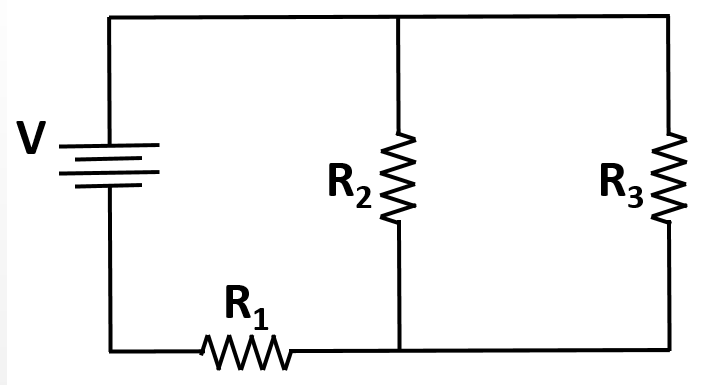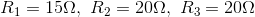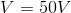What is the current delivered to the curcuit by the battery?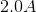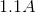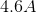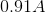Explanation:

Using Ohm's law to solve for the value of the current from the battery requires calculation of the equivalent resistance of the circuit.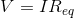The resistors R2 and R3 are in parallel with one another. Once combined, their requivalent resistor (R23) is in series with R1.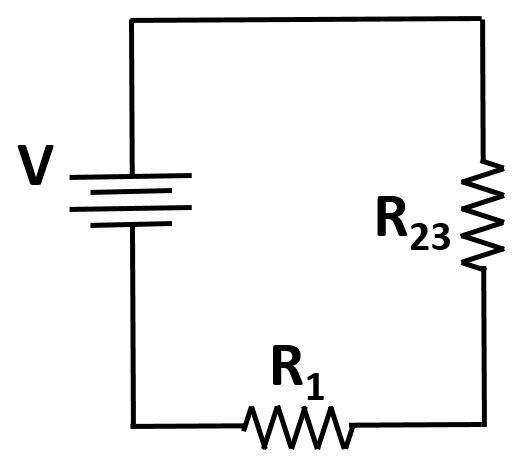The equivalent resistance is thus given by: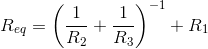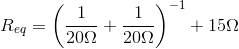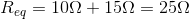Use this value and the given voltage to solve for the current in the circuit: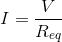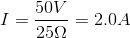### Example Question #1 : Circuit Relationships

A straight copper wire has a fixed voltage applied across its length. Which of the following changes would increase the power dissipated by this wire?

Changing the material of the wire to a metal of greater resistivity

Decreasing the cross-sectional area of the wire

Increasing the temperature of the wire

Decreasing the length of the wire

None of these options result in increased power dissipation

Decreasing the length of the wire

Explanation:

Relevant equations: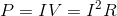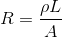Current and resistance are inversely proportional to one another, assuming voltage is fixed. Since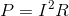, changes in current effect the power more than changes in resistance do. Thus, we need current to increase, meaning that resistance must decrease.

To decrease resistance, we could:

1. Change the material of the wire to one of lesser resistivity

2. Decrease the length of the wire

3. Increase the cross-sectional area of the wire

4. Decrease the temperature of the wire (very slight effect on resistance)

### Example Question #20 : Electric Circuits

A simple circuit contains two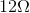resistors in parallel, connected to a 20V source. What power is being provided by the source to the circuit?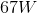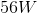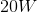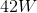Explanation:

The power supplied to the circuit can be calculated using the equation: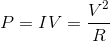To use this equation, we need to find the equivalent resistance of the circuit. Use the equation for equivalent resistance in parallel: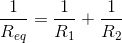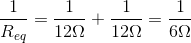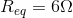Now that we have the resistance and the voltage, we can solve for the power.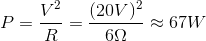### Example Question #21 : Electric Circuits

A battery is measured to have a potential of 5V. When connected to a wire with no resistors or other components, the voltage measured is 4.9V.

If the current through the wire is measured to be 2A, how much thermal energy is being lost per second as soon as the wire is connected to the battery?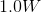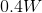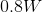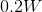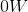Explanation:

First, we must know that the wire has some internal resistance. To calculate this, we need to know the potential drop through the wire, which must be the difference we saw from the initial voltage reading to the second. This value, 0.1V, we plug into Ohm's law to calculate the resistance of the wire.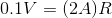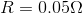The question asks for energy lost per second; this value is equivalent to the power.Use our values to solve.